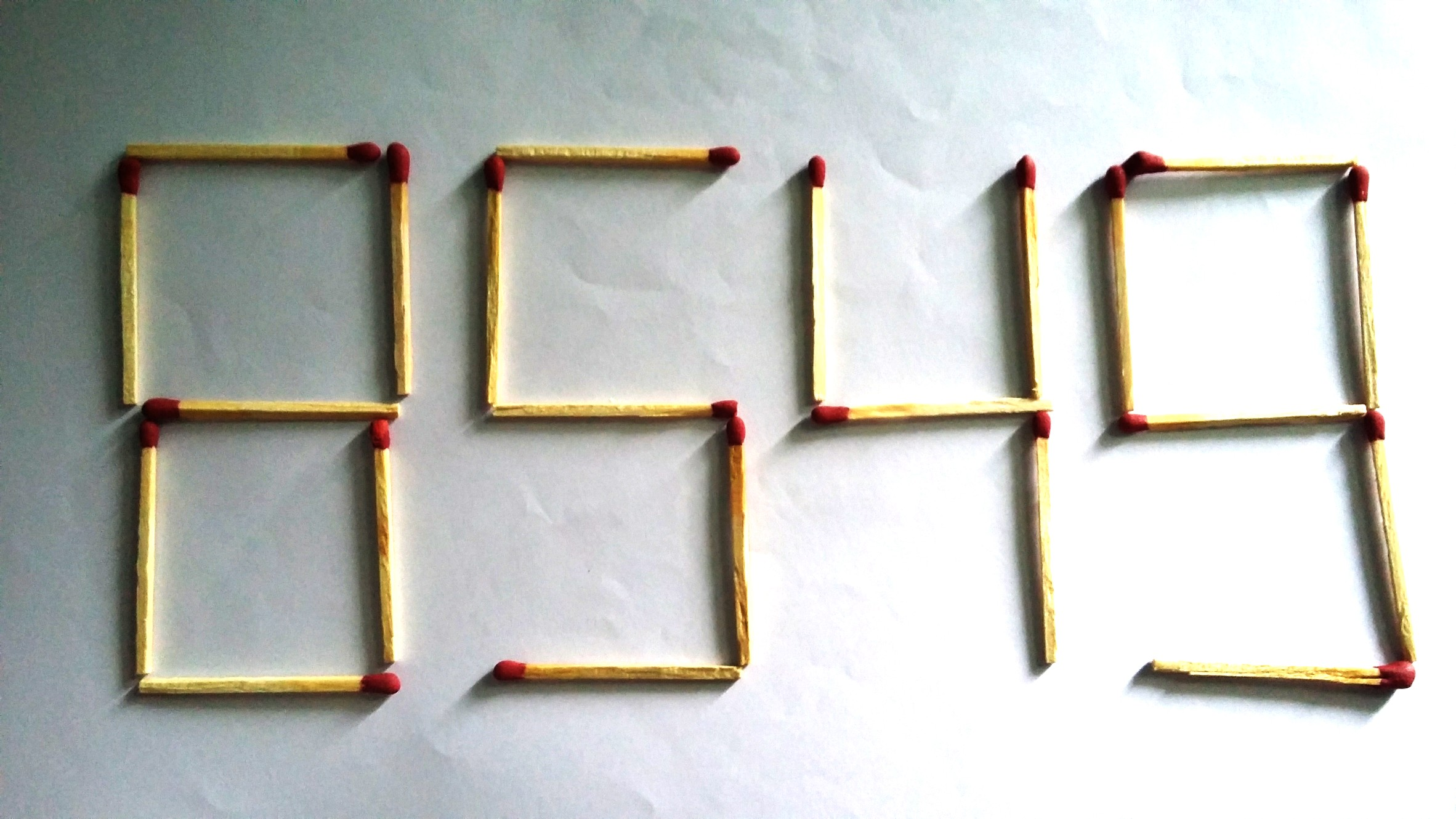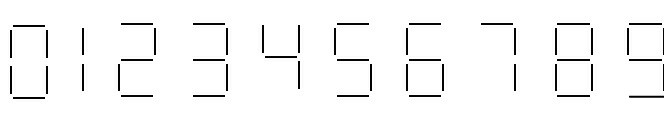# Matchstick Problem - 4The number 8549 shown in the picture above is formed by 22 matchsticks.

If you can shift exactly 2 matchsticks to another position, then what will be the $\color{#69047E} {\text {smallest}}$ $\color{#69047E} {\text{square}}$ of the positive integers you can make?

As an explicit example, suppose you can make 1000, 2000 & 3000, then the answer will be $\boldsymbol {1000 ^{2}=1000000}$

Details and Assumptions:

• All kinds of trick out of mathematics such as breaking or burning the matchsticks are not allowed.

• The digits formed by the matchsticks should be like the LED digits commonly used in calculators & LED watches. Here is an image you can follow:Warning: This question is kind of troll. Read the rules carefully and think twice before you answer.

×